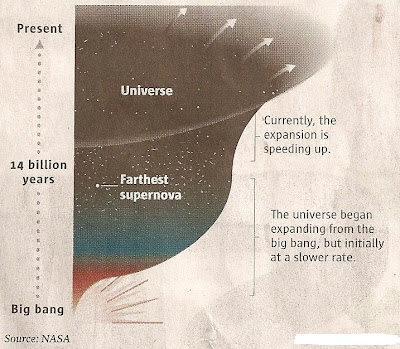## Wednesday, October 5, 2011

### Three Share Physics Nobel for Accelerated Cosmic Expansion DiscoveryThe recently announced awards for the Nobel Prize in Physics should come as no surprise to those who've been following events in cosmology the past two decades or so. That is, the universe has been found to be undergoing an accelerated rate of cosmic expansion. To put it into colloquial terms, the universe has been found to be not only stretching but stretching at a faster and faster rate.

The Prize itself was shared between Saul Perlmutter, of the Supernova Cosmology Project of the University of California Berkeley, who received half (see photo) and Brian Schmidt of the High-Z Supernova Search team at the Australian National Observatory, and Adam Riess of the Space Telescope Science Institute in Baltimore, MD. Both Riess and Schmidt shared the other half of the Nobel. All three worked in competition with each other.

Perlmutter's basic data graph, with the absolute magnitude (M) plotted against redshift (z) is shown in the graph adjacent to his picture above. Type Ia supernovae were selected because they all have a similar amount of mass so release energy at about the same rate ("luminosity") hence exhibit the same brightnesses. Thus, difference in brightness (as we saw with the distance modulus exercises in 'Tackling Simple Astronomy Problems') enables one to compute the distances.

In addition, by measuring the extent of the red shift, e.g.:

[L' - L]/ L = v/c

where L' denotes red-shifted wavelength, L the normal wavelength, c, the speed of light, it is possible to estimate how fast the supernova is receding (v).

In Perlmutter's case, it took four months of checking and re-checking his results before he accepted that, indeed, the rate of expansion was accelerating. In terms of the real data points, those associated with the Type Ia supernovae all fall to the LEFT and above the dotted line (see graph), or in what we call the 'accelerating universe' region. On the other side of the diagonal dotted line is the "decelerating region".

In his beautifully done summary presenting his work (See, e.g. 'Supernovae, Dark Energy and the Accelerating Universe', Physics Today, April, 2003, p. 53) Perlmutter not only shows the accelerating data but also provides an explanation in terms of dark energy. Thus, Perlmutter proposes dark energy as the primary agency for the ongoing increased rate of accelerated expansion.

He does this (ibid.) by first associating the "accelerating region" with what he calls "vacuum energy". He then invokes a cosmological "equation of state" (think of something like the equation of state for an ideal gas, e.g. P = nkT) for this vacuum energy, as:

w = (p/ rho) = -1

where p denotes pressure and rho energy density.

If: p less than (rho /3) we have gravity that repels

Or (If we set: 0 = (rho + 3p))

p = (- rho)/3

This is consistent with Einstein's theory of general relativity - which one could say approaches the status of a 'basic law of physics'. In this case, the existence of a negative pressure is consistent with general relativity's allowance for a "repulsive gravity" - since any negative pressure has associated with it gravity that repels rather than attracts.

Of course, simple algebra applied to the above also shows that the energy density would have to be negative, e.g. energy density = - (pressure).

These results all tossed the orthodox expectation that the universe would eventually re-collapse onto its head. Obviously, it can't re-collapse if it is forever accelerating with outwermost galaxy clusters growing further and further apart. For gauging this one uses the Omega parameter:

OMEGA = rho/ rho(c)

where rho(c) denotes a critical energy density.

If then rho > rho (c)

the universe would be able to re-collapse, else not.

Current data, e.g. from Boomerang and other satellite detectors shows that :

rho = 0.3 (rho (c))

I.e. that rho less than rho(c)

so there is no danger of the cosmos decelerating.

When the predictions of the different theoretical models are combined with the best measurements of the cosmic microwave background, galaxy clustering and supernova distances, we find that the proportion of the dark energy component of OMEGA:

0.62 < OMEGA_dark < 0.76,

where OMEGA_dark = rho_dark/ rho(c), and -1.3 < w < -0.9

Or to put in into the words of the Royal Swedish Academy of Sciences:

"If the expansion will continue to speed up the universe will end in ice"

Indeed!